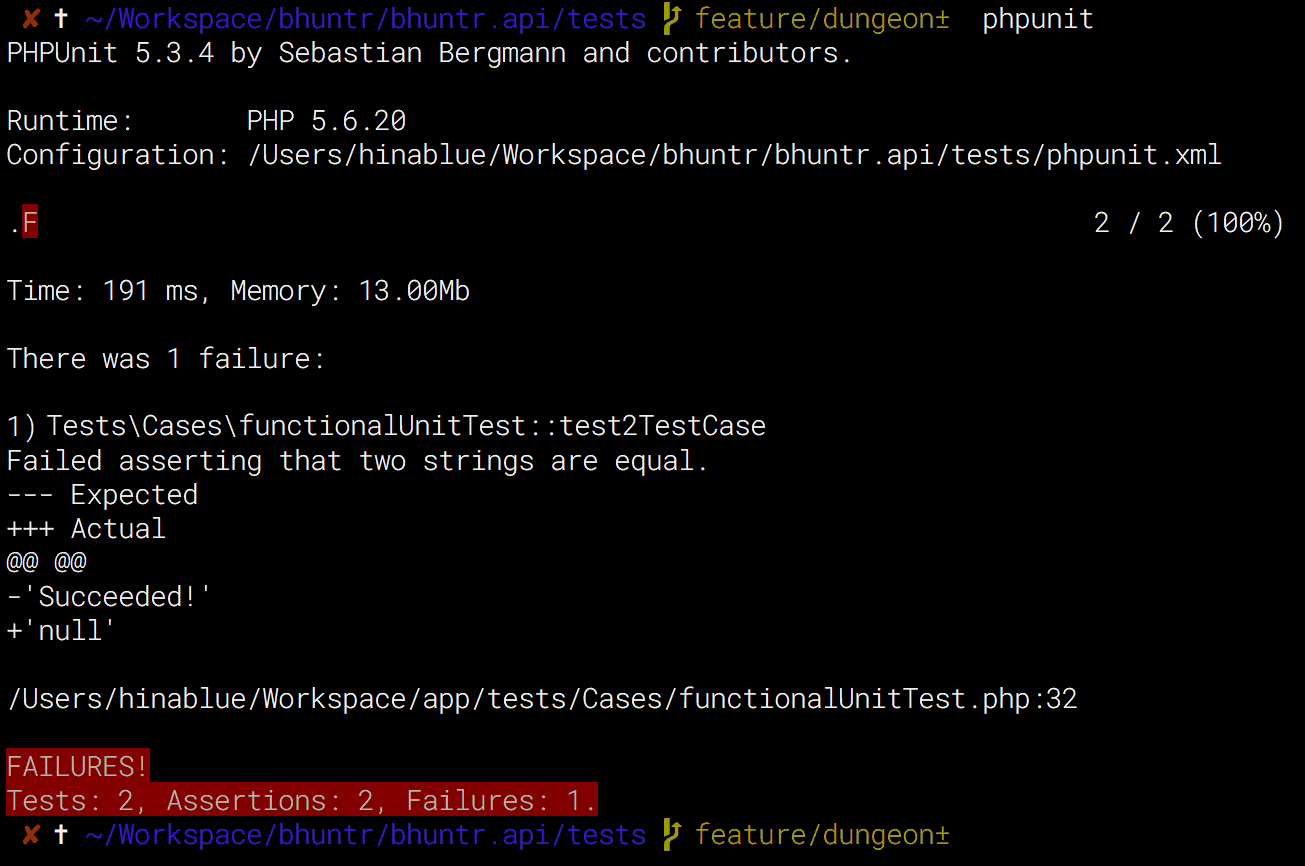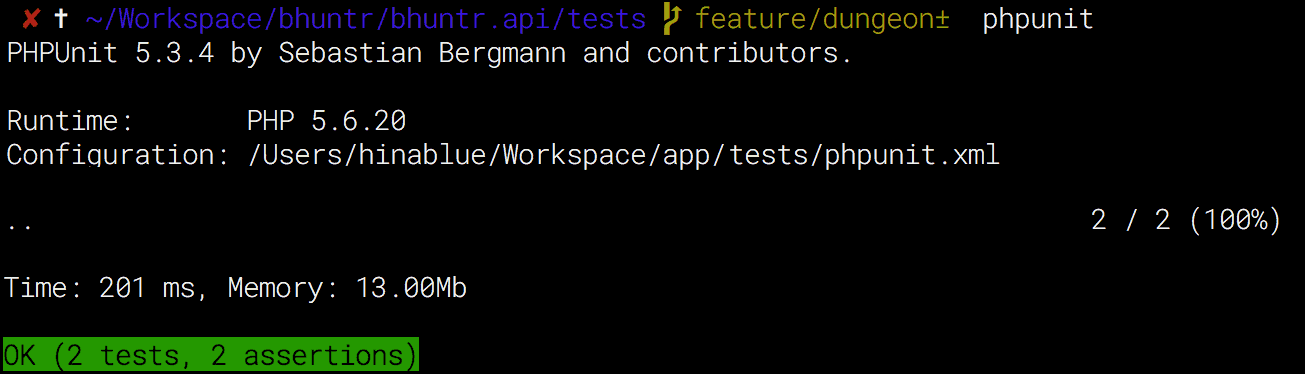## PHPUnit Mock## Mock with DI

Phalcon 有很多東西可以放在 DI 裡面，而，當我們要做 Functional Test 的時候，有些東西就必須要額外的傳入，舉個例子來說，

public function indexAction() {
// 我想要拿到 Request 的 get 傳入值
$params =$this->request->get();
return 'Succeeded!';
}


request 的來源是 \Phalcon\Http\Request 他原本就已經存在於 \Phalcon\Di\FactoryDefault() 裡面，所以本來是不需要額外設定。但是，因為你要跑的測試環境是在 CLI 底下，所以這個 request 就必須要用 Mock 來生一個替身並改寫，所以，理論上我們可以在 setUp() 裡面這樣做，

protected function setUp() {
$mock =$this->getMock('\\Phalcon\\Http\\Request', ['get']);
$mock->expects($this->any())
->method('get')
->will($this->returnValue('Succeeded!'));$this->di->setShared('request', $mock); }  那我們寫兩段測試， public function test1TestCase() {$response = $this->dispatch('/index');$this->assertEquals('Succeeded!', $response); } public function test2TestCase() {$response = $this->dispatch('/index');$this->assertEquals('Succeeded!', $response); }  最終執行結果會是，疑？如果 Mock 有順利 Inject 到 $app 的話，理論上應該會拿到兩次都是成功的回應才對？為什麼第二次是 NULL 的結果？

->will($this->returnCallback(array($this, 'getMockReturnValue')));

• 只要有任何一個 TestCase 通過，這個 Mock 就會被消滅。
• 連帶的已經被埋入 DI 的 request 也會變回自己（而不是 替身
• 反之測試要是 不通過，這個 Mock 就會繼續存活下去，直到有任何人通過測試。

1. 我每次 setUp 都重新設定 Mock 並且重新注入 DI
2. 即便是把 \Phalcon\Http\Request 拿去外面重新 extends 再做 Mock 一樣失敗
3. 是否是 PhalconPHP 哪裡做了什麼事情？
4. 或是 PHPUnit Mock 不能這樣做？

## Functional Test 帶入自訂資料參數

1. 寫一個 Class 叫做 mockRequest
2. extends \Phalcon\Http\Request
3. 抄一下 Phalcon 原始碼關於 getJsonRawBody, get 的部分
4. 如果你用到更多，請多抄點
5. 抄完記得改
6. 加一個方法 setRequest 讓我們可以傳自訂資料進來
7. $this->di->setShared('request', new mockRequest()); 8. 在 Dispatch 的時候設定參數 9. 收工 那個 Class 大概是長這樣， class mockRequest extends \Phalcon\Http\Request { protected$mock_params = [];

public function setRequest(array $params = []) {$this->mock_params = $params; } public function getJsonRawBody($convert = false) {
if (true === $convert) { return$this->mock_params;
}
return json_encode($this->mock_params); } public function get($name = null, $filters = NULL,$defaultValue = NULL) {
if (!is_null($name)) { if (isset($this->mock_params[$name])) { return$this->mock_params[$name]; } else { return null; } } return$this->mock_params;
}
}


function dispatch($url,$params) {
$params = array_merge($params, [
'key' => '你抓不到我',
'cm' => 30
]);
$request =$this->di->getShared('request');
$request->setRequest($params);

// ... 以下省略.
}